# oracle sql 高级编程学习笔记（十五）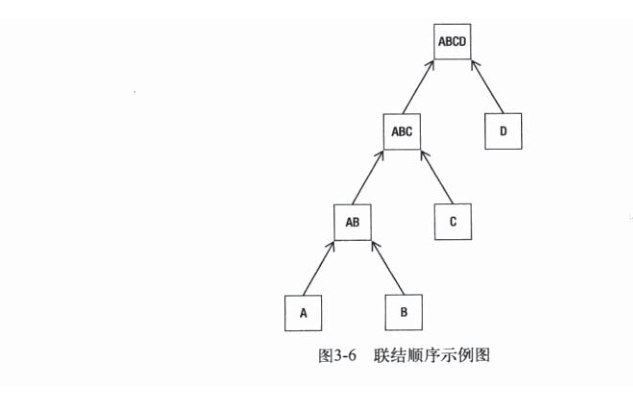# 一、嵌套循环联结

（与散列联结对应）嵌套循环：一般小表为驱动表，大表为被驱动表，由小表中满足条件的数据去大表中循环遍历查找满足条件的数据。一般来说大表的条件列上有索引会加快查询速度。小表被查询一次，大表扫描N次。

/*+ leading(dept) use_nl(emp)*/ 强制使用循环嵌套联结
select  /*+ leading(d) use_nl(e)*/ e.ename,d.deptno,d.dname ,d.loc from  emp e ,dept  d where e.deptno=d.deptno;



 for each row in( select ename,deptno from emp) loop
for (select dname,loc  from dept where deptno=outer.deptno) loop
if match then pass the row on to the next step
if inner join and  no match then discart the row
if outer join and no match  set inner  column values to null
and pass the row on to  the next step
end loop
end loop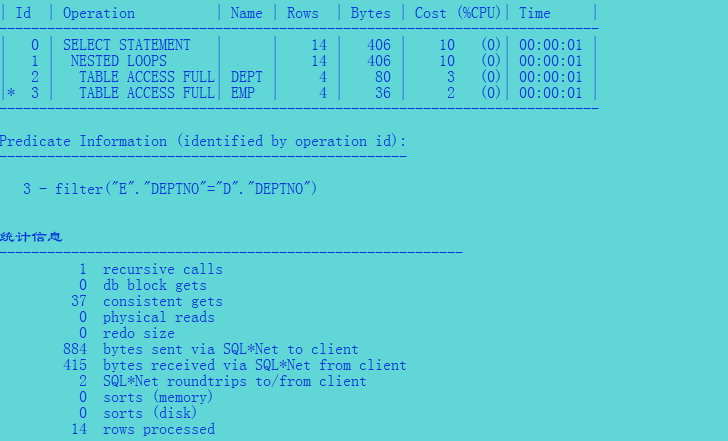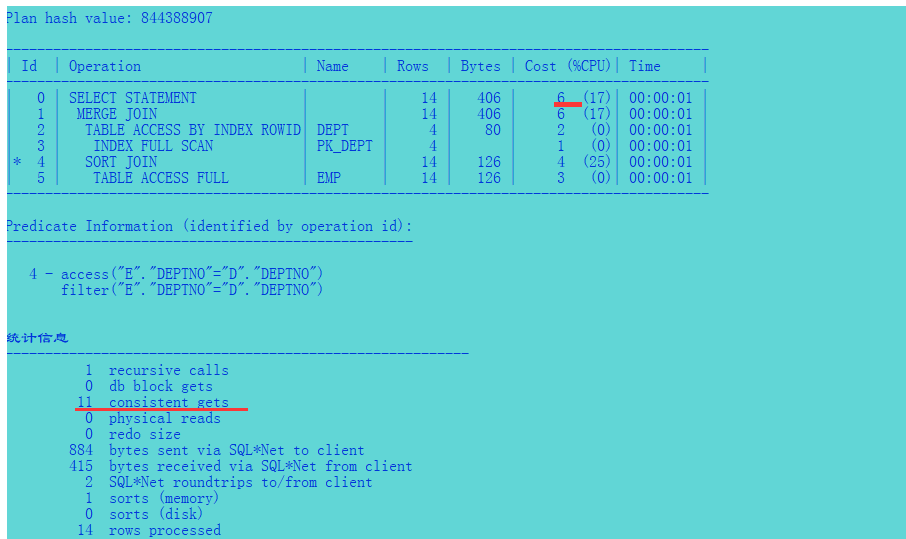# 二、排序-合并联结


select e.ename,d.deptno,d.dname ,d.loc from  emp e ,dept  d where e.deptno=d.deptno;


 select empno，ename, deptno from emp order by deptno
select dname,loc,deptno from dept order by deptno
compare the rowsets and return rows where deptno  in both lists match
for an outer join ,compare the rowsets and return allrows from  the first
list  setting column values for the other table to null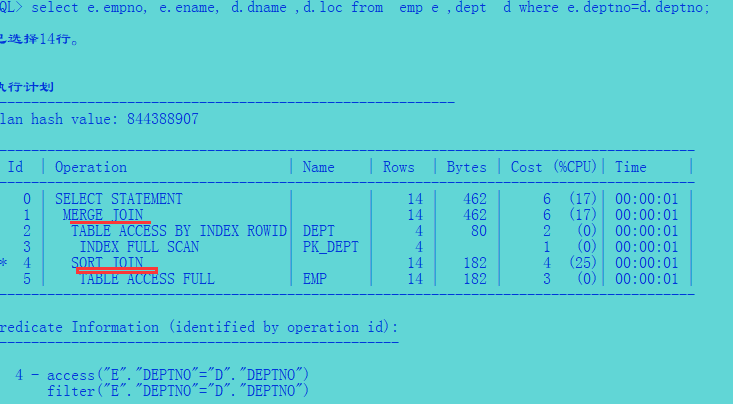# 三、散列联结

 select  /*+ leading(e) use_hash(d)*/ e.ename,d.deptno,d.dname ,d.loc from  emp e ,dept  d where e.deptno=d.deptn


determine the smaller row set ，or in the case of an outer join
use the outer joined table
select  deptno,dname,loc  from dept
hash the deptno column  and build a hash table
select ename ,deptno from  emp
hash the deptno column  and probe the hash table
if  match made ,check bitmap to confirm row match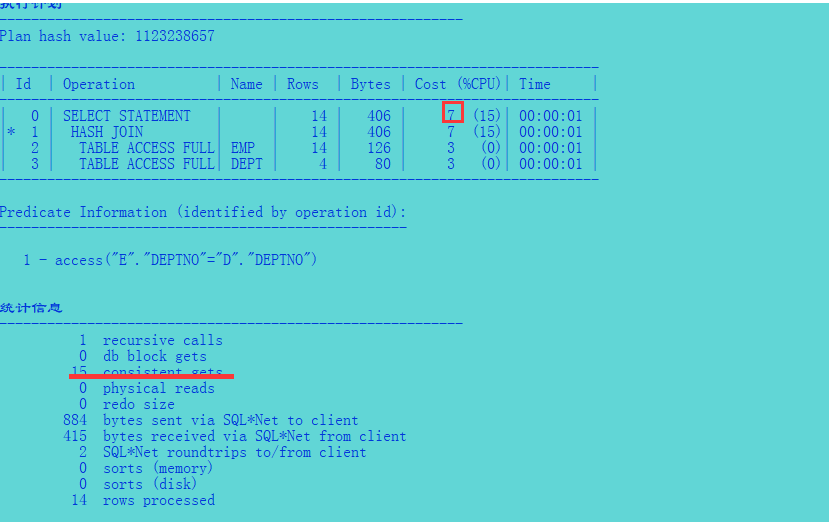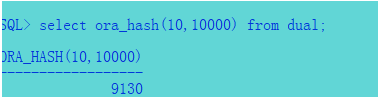create table emp_tmp(e_id number(6),gender varchar2(2));
create table loc_tmp(e_id number(6),loc varchar2(10));
begin
for  i in 1..5000 loop
insert into  emp_tmp values(i,'F');
END loop;
commit;
end;
begin
for  i in 1..5000 loop
insert into  loc_tmp values(i,'KM'||i);
END loop;
commit;
end;
alter table loc_tmp add primary key     (loc);
select e.e_id,l.loc from   emp_tmp e ,loc_tmp l
where e.e_id=l.e_id;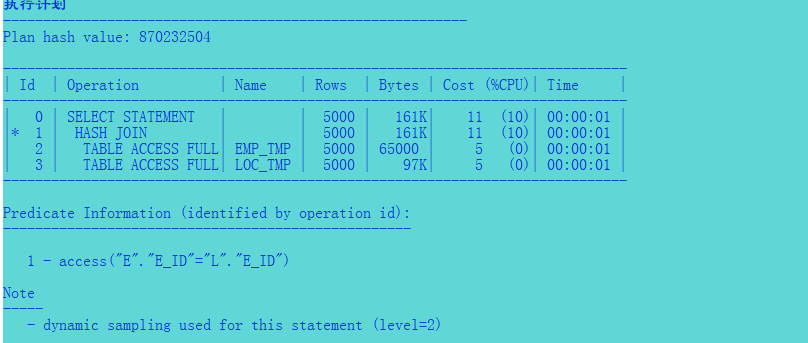# 四、外联结

Oracle 使用’+’ 字符来表明进行外联结。

select  c.cust_last_name ,nvl(sum(o.order_total),0) tot_orders from   customers c ,orders o
where c.customer_id=o.customer_id
group by  c.cust_last_name
having nvl(sum(o.order_total),0) between 0 and 5000
order by c.cust_last_name;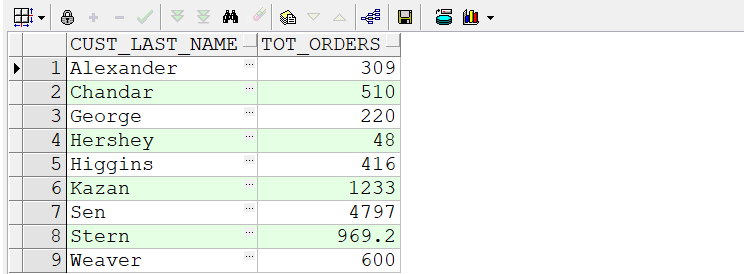select  c.cust_last_name ,nvl(sum(o.order_total),0) tot_orders from   customers c ,orders o
where c.customer_id=o.customer_id(+)
group by  c.cust_last_name
having nvl(sum(o.order_total),0) between 0 and 5000
order by c.cust_last_name;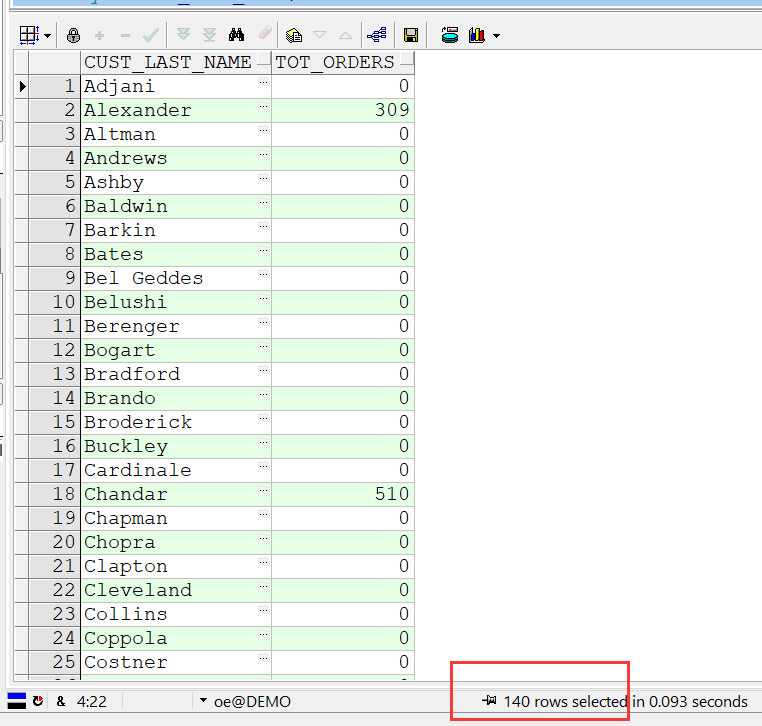ANSI 外联结的相同语法

select  c.cust_last_name ,nvl(sum(o.order_total),0) tot_orders from   customers c left outer join orders o
on  c.customer_id=o.customer_id
group by  c.cust_last_name
having nvl(sum(o.order_total),0) between 0 and 5000
order by c.cust_last_name;